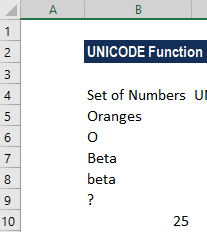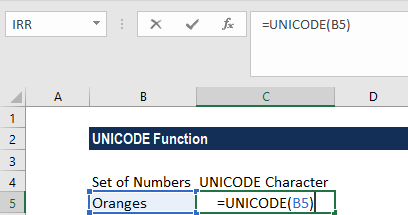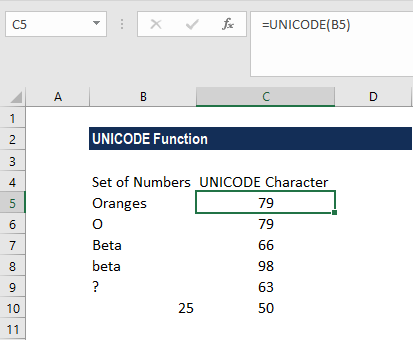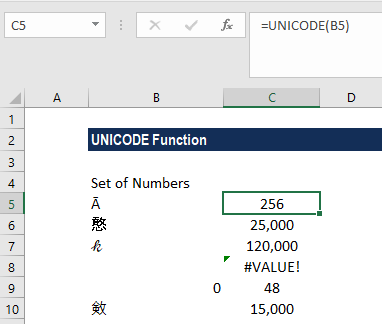# UNICODE Function

Returns the code point for the first character of a supplied text string

## What is the UNICODE Function?

The UNICODE Function is categorized under Excel Text functions. It will give the number (code point) for the first character of a supplied text string. The function was introduced in MS Excel 2013.

### Formula

=UNICODE(text)

The UNICODE Function requires the following argument:

1. Text (required argument) – This is the character or text string for which we need the Unicode character. The text argument can be a single character that is surrounded by double quotation marks (” “) entered directly into the function or a cell reference to the location of the character in a worksheet.

### How to use the UNICODE Function in Excel?

To understand the uses of the UNICODE function, let’s look at a few examples:

#### Example 1

Suppose we are given a set of data that we wish to convert to UNICODE characters. First, we will list down the data in a column, as shown below:In the adjacent column, we will enter the following formula:We get the results below:1. When we gave Oranges, the UNICODE function returned the code point for the character “O”. It gives the same result for UNICODE (“O”), which is 79.
2. When we gave Beta, the UNICODE function returned the code point for the character “B” (UPPERCASE). The result is 66.
3. When we gave beta, the UNICODE function returned the code point for the character “b” (lowercase). The result is 98.

#### Uppercase vs. Lowercase Letters

Remember that the uppercase or capital letters on the keyboard use different character codes than the corresponding lowercase or small letters.

For example, the UNICODE/ANSI code number for the uppercase “A” is 65 while for the lowercase “a”, the UNICODE/ANSI code number is 97.

#### Example 2

Let’s now see how the function converts characters. Using UNICODE, we can translate the characters into numbers, as shown below:We got a #VALUE! error because we gave an unidentified number in row 8.

### Few pointers about the UNICODE Function

1. #VALUE! error – Occurs when:
• Unicode numbers are partial surrogates and data types are not valid.
• Unicode numbers fall outside the range allowed.
2. The UNICODE function is the opposite of the UNICHAR function.

Thanks for reading CFI’s guide to important Excel functions. By taking the time to learn and master these functions, you’ll significantly speed up your financial analysis. To learn more, check out these additional CFI resources:

• Excel Functions for Finance